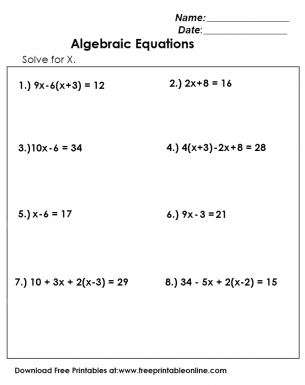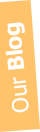# Algebraic Equations - Solve These Equations - Free Algebraic Math Worksheets for Middle School

 Algebraic Equations are univariate, which means that it involves only one variable. Learn how to solve them. Click on the template to Download now for Free!# Views: 2893 | # Downloads: 46 | # Prints: 166 Click for Printing Tips

By: Jenny Printable

Algebra, a crucial aspect of mathematics, introduces the principle of using letters and symbols to represent numbers and their relationships in equations.

## The Basics of Algebraic Equations

Algebraic equations comprise both constants (like 2, -7, or 5) and variables (like x, y, or z), brought together by mathematical operations.

The goal is to find the value of the variable that makes the equation true. These equations are the stepping stone to understanding more complex mathematical concepts in later grades.

Algebra acts as the gateway to advanced mathematics and is used extensively in fields like Engineering, Medicine, and Economics. Mastering algebra equips students with problem-solving skills, logical reasoning, and the ability to think abstractly.

Our free worksheets are tailored for middle school students to assist in understanding and mastering Algebraic equations.

They offer a mix of easy and challenging problems to keep the students engaged while gradually enhancing their skills. With solutions provided, students can practice at their own pace, ensuring a solid foundation in Algebra.

Explore, download, and solve these worksheets. Let’s instill a passion for Algebra and build a stepping stone for future mathematical endeavours.

We offer free printable worksheets for different levels that include exercises that tackle grammar and language.

Our collection of free printable worksheets is perfect for your kids at different grade levels.